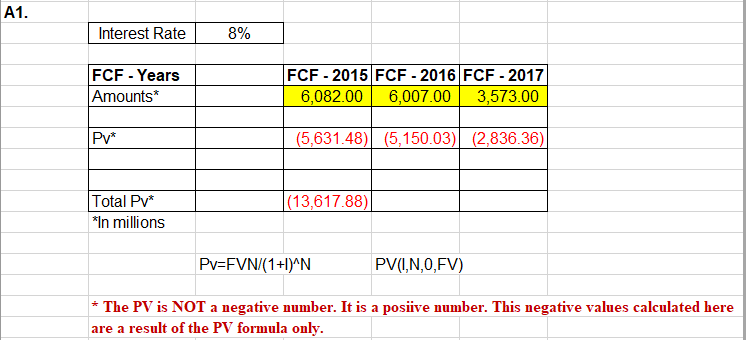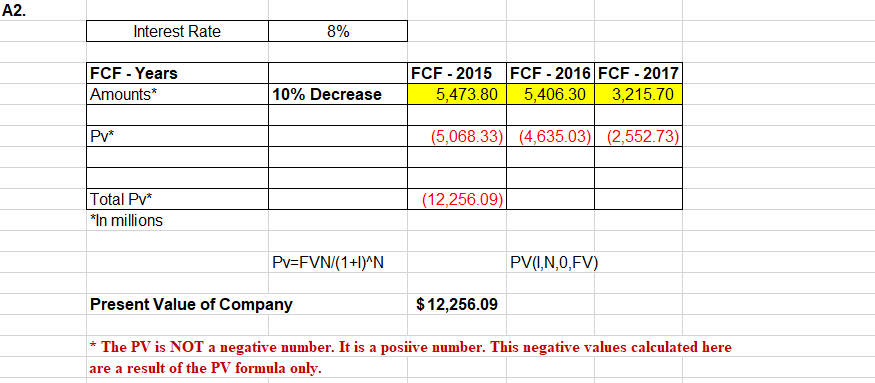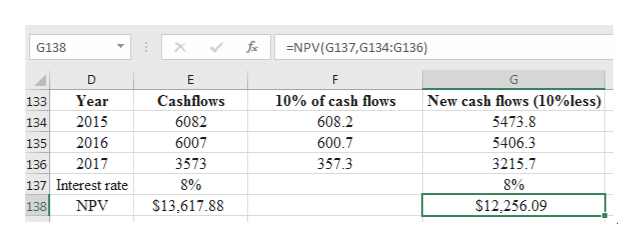# A1.Interest Rate8%FCF - 2015 FCF -2016 FCF -20176,082.00FCF - YearsAmounts*6,007.003,573.00Pv*(5,631.48)(5,150.03) (2,836.36)Total Pv*|(13,617.88)*In millionsPv=FVN/(1+I)^NPV(,N,0,FV)The PV is NOT a negative number. It is a posiive number. This negative values calculated hereare a result of the PV formula only A2.Interest Rate8%FCF - YearsAmounts*FCF - 2015 FCF - 2016 FCF - 20175,406.3010% Decrease3,215.705,473.80(5,068.33) (4,635.03) (2,552.73)Pv*(12,256.09)Total Pv*In millionsPv=FVN/(1+I^NPV(I,N,0,FV)Present Value of Company\$12,256.09*The PV is NOT a negative number. It is a posiive number. This negative values calculated hereare a result of the PV formula only

Question
1 views

Suppose the risk of the company changes based on an unanticipated decrease in the Free Cash Flows by 10% annually during the years 2015, 2016, and 2017.

What are the implications of the change in present value based on risk? In other words, what does the change mean to the company, and how would a financial manager interpret it?help_outlineImage TranscriptioncloseA1. Interest Rate 8% FCF - 2015 FCF -2016 FCF -2017 6,082.00 FCF - Years Amounts* 6,007.00 3,573.00 Pv* (5,631.48)(5,150.03) (2,836.36) Total Pv* |(13,617.88) *In millions Pv=FVN/(1+I)^N PV(,N,0,FV) The PV is NOT a negative number. It is a posiive number. This negative values calculated here are a result of the PV formula only fullscreenhelp_outlineImage TranscriptioncloseA2. Interest Rate 8% FCF - Years Amounts* FCF - 2015 FCF - 2016 FCF - 2017 5,406.30 10% Decrease 3,215.70 5,473.80 (5,068.33) (4,635.03) (2,552.73) Pv* (12,256.09) Total Pv* In millions Pv=FVN/(1+I^N PV(I,N,0,FV) Present Value of Company \$12,256.09 *The PV is NOT a negative number. It is a posiive number. This negative values calculated here are a result of the PV formula only fullscreen
check_circle

Step 1

The present value is calculated today to determine the present value of future cashflows at the given discount rate.

Step 2

Given:

Interest rate is 8%

Future cash flows for three years are as follows:

Step 3

Explanation:

The change in NPV after 10% dec...help_outlineImage TranscriptioncloseG138 NPV(G137,G134:G136) E F G New cash flows (10%less) 5473.8 Year Cashflows 10% of cash flows 133 2015 6082 608.2 134 2016 600.7 6007 5406.3 135 357.3 2017 3573 3215.7 136 137 Interest rate 8% 8% NPV \$12,256.09 \$13,617.88 138 fullscreen

### Want to see the full answer?

See Solution

#### Want to see this answer and more?

Solutions are written by subject experts who are available 24/7. Questions are typically answered within 1 hour.*

See Solution
*Response times may vary by subject and question.
Tagged in